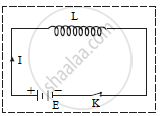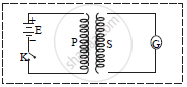HSC Science (General) 12th Board ExamMaharashtra State Board
Share

Explain Self Induction and Mutual Induction - HSC Science (General) 12th Board Exam - Physics

Question

Explain self induction and mutual induction

Solution

Derivation of induced e.m.f due to self induction:a. Consider a coil connected with battery E, plug key K and inductor L carrying current of magnitude I as shown in figure.
b. Since magnetic flux linked with the coil is directly proportional to the current.

phi prop I

phi prop LI...................(1)

where, L = constant called coefficient of self induction or self inductance of the coil, which depends upon the material of the core, number of turns, shape and area of the coil

c. Induced e.m.f in the coil is given by,

e=-(dphi)/dt

e=-L(dI)/dt.....................(2)

-ve sign in equation (ii) shows that self induced e.m.f opposes the rate of change of current.

|e|=|-L(dI)/dt|=L(dI)/dt

therefore Magnitude of self induced e.m.f is given by, |e|=L(dI)/dt

This is required induced e.m.f.

Derivation of induced e.m.f. due to mutual induction:a. Consider primary coil P and secondary coil S fitted with galvanometer G are placed very close to each other as shown in figure. The coil P is connected in series with the source of e.m.f (battery) and key K.
b. When tap key K is pressed current IP passes through the coil P. Magnetic flux phi_S linked with secondary coil S at any instant is directly proportional to current IP through primary coil P at that instant.

phi_s prop I_p

phi_s=MI_p........................(1)

where M is constant called coefficient of mutual induction or mutual inductance of the
coil.

e.m.f induced in S at any instant is given by, e_s=-(dphi_s)/dt

=-d/dt(MI_p)

e_s=-M(dI_p)/dt

Magnitude of induced e.m.f is given by,

|e_s|=-|M(dI_p)/dt|=(MdI_p)/dt

M=e_s/((dI_p)/dt)

Is there an error in this question or solution?

APPEARS IN

2016-2017 (March) (with solutions)
Question 7.2 | 3.00 marks

Video TutorialsVIEW ALL 

Solution Explain Self Induction and Mutual Induction Concept: Inductance - Mutual Inductance.
S